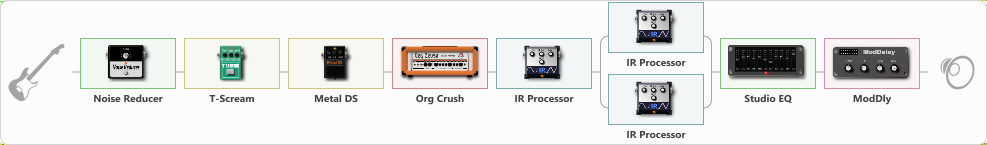# Van Halen

Discussion in 'ToneLib-GFX presets' started by Tony the Metalhead, Dec 5, 2021.

1. ### Tony the MetalheadActive Member

Van Halen

Preset name: E V H

Effects chain:Effect: "Noise Reducer" (Dynamics / Filter), active - "yes"
{
"Sens" = 77
"Mode" = Hard
}

Effect: "T-Scream" (Overdrive / Distortion), active - "yes"
{
"Drive" = 30
"Tone" = 60
"Level" = 10
}

Effect: "Metal DS" (Overdrive / Distortion), active - "yes"
{
"Dist" = 80
"Bass" = 20
"Middle" = 10
"Treble" = 40
"Level" = 40
}

Effect: "Org Crush" (Amp simulators), active - "yes"
{
"Gain" = 80
"Bass" = 25
"Middle" = 20
"Treble" = 40
"Presence" = 85
"Master" = 30
"Level (dB)" = 6
}

Effect: "IR Processor" (Cabinets), active - "yes"
{
"IR" = Van Halen Van Halen
"Low Cut (Hz)" = 0
"Hi Cut (kHz)" = 20.0
"Mix" = 100
"Level (dB)" = 0
}

Effect: "Splitter" (Dynamics / Filter)
{
"A-Bypass" = Off
"A-Pan" = 0
"A-Level" = 55
"B-Bypass" = Off
"B-Pan" = 0
"B-Level" = 55

'A' branch:
{

Effect: "IR Processor" (Cabinets), active - "yes"
{
"IR" = CT_ED3412EVH_57
"Low Cut (Hz)" = 100
"Hi Cut (kHz)" = 20.0
"Mix" = 100
"Level (dB)" = 0
}
}
'B' branch:
{

Effect: "IR Processor" (Cabinets), active - "yes"
{
"IR" = CT_ED3412EVH_57
"Low Cut (Hz)" = 80
"Hi Cut (kHz)" = 20.0
"Mix" = 100
"Level (dB)" = 0
}
}
}

Effect: "Studio EQ" (Dynamics / Filter), active - "yes"
{
"31 Hz" = -2
"62 Hz" = -3
"125 Hz" = -4
"250 Hz" = -7
"500 Hz" = -8
"1 kHz" = -5
"2 kHz" = -4
"4 kHz" = -5
"8 kHz" = -4
"16 kHz" = 10
"Level (dB)" = 0
}

Effect: "ModDly" (Delay), active - "yes"
{
"Time" = 250
"Feedback" = 25
"Tone" = 50
"Speed" = 1.5
"Mix" = 25
}

Note: You will need to download and install the ToneLib-GFX software to use the preset.

File size:
19.1 KB
Views:
2,623